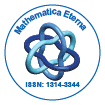Mathematica EternaOpen Access

ISSN: 1314-3344+32-466902147

Abstract

Some mathematical issues with MATLAB

Sahem Tarawneh, Eman Al-Sarairah and Feras Al Faqih

The paper must have abstract not exceeding 200 words. In this paper, we shall investigate some mathematical difficulties that is facing learners and undergraduates in science and engineering when they use MATLAB. When solving some mathematical problems using this software, one may unfortunately, face the following: no answer at all, wrong answer, long and complicated answer. Therefore, our main aim is to investigate such problems through mathematical examples in order for the user to be aware of.

Top